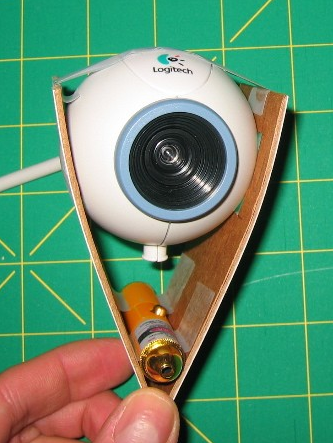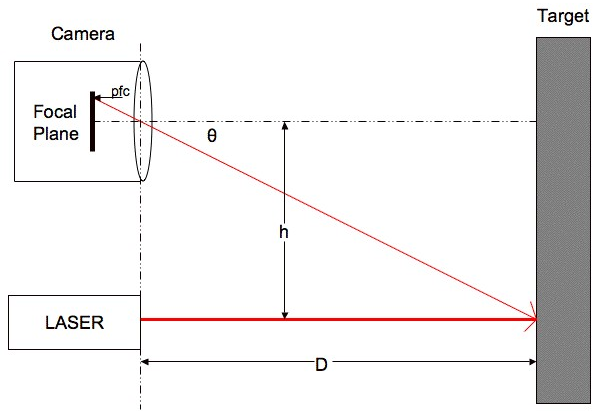$D=h/tan(\theta)$

$\theta=pfc*rpc+ro$

$$pfc$$ ＝从焦平面到中心的像素数量
$$rpc$$ ＝单个像素的弧度
$$ro$$ ＝弧度补偿（弥补对齐错误）

$D=frac{h/tan(pfc*rpc+ro)}$

 校正数据 pixels from center actual D (cm) 103 29 81 45 65 58 55 71 49 90 45 109 41 127 39 159 37 189 35 218

$\theta_(actural)=arctan(h/D_(actural))$

$$\theta_(actural)$$＝真实角度
$$D_(actural)$$＝真实距离（测量得出）

$D=frac{h/tan(pfc*rpc+ro)}$

 实际和计算的测量数据 pixels from center calc D (cm) actual D (cm) % error 103 29.84 29 2.88 81 41.46 45 -7.87 65 57.55 58 -0.78 55 75.81 71 6.77 49 93.57 90 3.96 45 110.85 109 1.70 41 135.94 127 7.04 39 153.27 159 -3.60 37 175.66 189 -7.06 35 205.70 218 -5.64

### 一条评论

1.过来帮顶了，了解一下，赞